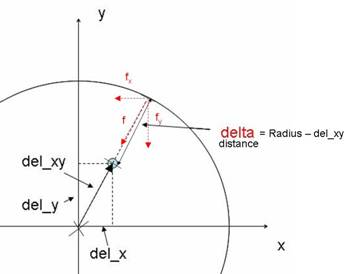# [lammps-users] three questions of wall_lj126

Dear ALL,

I have three questions of wall_lj126.

1.the main idea of adding the z-cylinder boundary to the fix_wall_lj126 command

1. the use of the “fwall” and the “wall”.

2. the use of “wall_flag”. Is it just for MPI flag?

Hope for any suggestions!!!

I want to add the z-cylinder boundary to the fix_wall_lj126 command. After perused the source code of fix_wall_lj126.cpp and fix_wall_lj126.h, I found that the key point is distributing the force f into fx and fy directions and then add them of the force which interact on the particle as the sketch shown below.int wallstyle; //to distinguish cylinder type from previous type.

double cylinder_X,cylinder_Y,cylradius; //to record the x and y axis and radius of the cylinder.

in fix_wall_lj126.h.

Am I right?

I felt that the force computation is located at the FixWallLJ126::post_force(int vflag). The exact part

for (int i = 0; i < nlocal; i++)

There are two punch-drunk parameters in this part. There are the “fwall” and the “wall”. Both of them have 4 components. Is it the first component is the overall value and the other there for x y z directions? Whether the “fwall” means the force from the wall and the “wall” means the potential? Why the fwall can be directly added to wall?

The original source is

for (int i = 0; i < nlocal; i++)

if (side == -1) delta = x[i][dim] - coord;

else delta = coord - x[i][dim];

if (delta <= 0.0) continue;

if (delta > cutoff) continue;

rinv = 1.0/delta;

r2inv = rinv*rinv;

r6inv = r2invr2invr2inv;

fwall = r6inv*(coeff1*r6inv - coeff2) * side;

f[i][dim] -= fwall;

wall += r6inv*(coeff3*r6inv - coeff4) - offset;

wall[dim+1] += fwall;

}

So I modifid that

/*

if(wallstyle==1)

{

double del_x,del_y,del_xy;

del_x=del_y=del_xy=0;

double fwall_x,fwall_y;

fwall_x=fwall_y=0;

for (int i = 0; i < nlocal; i++)

{

{

del_x=(x[i]-cylinder_X);

del_y=(x[i]-cylinder_Y);

del_xy=sqrt(del_xdel_x+del_ydel_y);

if (delta <= 0.0) continue;

if (delta > cutoff) continue;

rinv = 1.0/delta;

r2inv = rinv*rinv;

r6inv = r2invr2invr2inv;

fwall = r6inv*(coeff1*r6inv - coeff2);

fwall_x= fwall*del_x/del_xy;

fwall_y = fwall*del_y/del_xy;

f[i] -= fwall_x;

f[i] -= fwall_y;

wall += r6inv*(coeff3*r6inv - coeff4) - offset;

wall += fwall_x;

wall += fwall_y;

}

}

}

*/